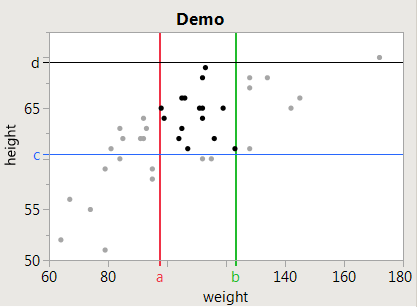The World Statistics Day celebration continues here in the Community. We all need reliable data for sound decision making. Do you have a data source that you trust most? Head over to Discussions to tell us about it.
Choose Language Hide Translation Bar
Highlighted

## Graph Builder - adding interactive reference lines

When using graph builder, it allows you to "highlight" or "select" a specific data subset within the legend. The corresponding output on the graph builder and data table will also highlight themselves. When a subset of data is selected, the other subsets fade into the background or increase their transparency in an interactive manner.

Can I have add reference lines that correspond to a selected data set behave interactively in the same way? And can I have these lines automatically color themselves as they do when I drop a nominal column into the legend field?

Highlighted

## Re: Graph Builder - adding interactive reference lines

Here's some starter JSL.

``````dt = Open( "\$SAMPLE_DATA/Big Class.jmp" );

oldselection = [];// don't update when nothing changes by comparing old to new

f = Function( {a}, // this callback tells which points are changing selected state
// and may send between 1 and N row numbers in the matrix a.
// this example will ignore a and just ask the table about the range of the currently
// selected points.
{subset, hmin, hmax, wmin, wmax, newselection}, // local variables
newselection = dt << getselectedrows; // compare to previous selection...
If( Try( N Rows( newselection ) != N Rows( oldselection ) | Any( newselection != oldselection ), 1 ),
oldselection = newselection; // remember the new selection for next time
dtSubset = dt << subset( SelctedRows( 1 ), LinkToOriginalDataTable( 1 ), invisible ); // make a subset of the selection to summarize
dtSummary = dtSubset << Summary( Min( :height ), Min( :weight ), Max( :height ), Max( :weight ), Freq( "None" ), Weight( "None" ) );
Close( dtSubset, "nosave" ); // done with the subset, use the summary...
hmin = dtSummary:name( "Min(Height)" ); // capture the range of the selected axis
hmax = dtSummary:name( "Max(Height)" );
wmin = dtSummary:name( "Min(Weight)" );
wmax = dtSummary:name( "Max(Weight)" );
Close( dtSummary, "nosave" ); // done with the summary, use the captured values...
// remove 2 old reflines first, from each axis, then add 2 new ones
// remove the old ref lines from the weight axis (1==horizontal)
Report( gb )[axisbox( 1 )] << removerefline( (Report( gb )[axisbox( 1 )] << getscript)["addrefline"] );
Report( gb )[axisbox( 1 )] << removerefline( (Report( gb )[axisbox( 1 )] << getscript)["addrefline"] );
// remove the old ref lines from the height axis (2==vertical)
Report( gb )[axisbox( 2 )] << removerefline( (Report( gb )[axisbox( 2 )] << getscript)["addrefline"] );
Report( gb )[axisbox( 2 )] << removerefline( (Report( gb )[axisbox( 2 )] << getscript)["addrefline"] );
// add the new ref lines...extend the bounding box by .5 for the integer height/weight (not required)
Report( gb )[axisbox( 1 )] << addrefline( wmin - .5, "solid", "red", "a", 2 );
Report( gb )[axisbox( 1 )] << addrefline( wmax + .5, "solid", "green", "b", 2 );
Report( gb )[axisbox( 2 )] << addrefline( hmin - .5, "solid", "blue", "c", 1 );
Report( gb )[axisbox( 2 )] << addrefline( hmax + .5, "solid", "black", "d", 1 );

);
);

rs = dt << make row state handler( f );

gb = Graph Builder(
Size( 416, 300 ),
Show Control Panel( 0 ),
Show Legend( 0 ),
Variables( X( :weight ), Y( :height ) ),
Elements( Points( X, Y, Legend( 3 ) ) ),
SendToReport( Dispatch( {}, "Graph Builder", OutlineBox, {Set Title( "" )} ), Dispatch( {}, "graph title", TextEditBox, {Set Text( "Demo" )} ) )
);

``````

The JSL installs a RowState handler function in the data table; that function is notified when the table's row state data changes (when a row is selected/hidden/excluded, or the marker style or color changes.) The function calculates the min and max values of the selected columns and removes old ref lines and adds new ref lines.The a-b-c-d ref lines are interactively tracking the rectangular point selection

It flashes as it updates because the display redraws when each refline is added or removed; it may get too slow with large data that takes too long to redraw the graph.

Craige
Article Labels

There are no labels assigned to this post.